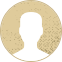Top Special Offer! Check discount

Get 13% off your first order - useTopStart13discount code now!

# Background of density-functional theory and plane-wave methodology

155 views 3 pages ~ 595 words
Get a Custom Essay Writer Just For You!

Experts in this subject field are ready to write an original essay following your instructions to the dot!

Density-functional theory and plane-wave approach background

Introduction

The density functional theory is made up of many models for simulation, including electronic, atomic, microstructural, and continuum domains. The electronic modeling is supported by the one-electron Schrödinger equation, from which an effective many-electron system can be generated.

Figure 1: Model Hierarchy

The Schrödinger equation is provided by

Where is the Hamiltonian operator represented in terms of kinetic energy and potential energy, V. (r) is the wave function, and the function is the energy.Expressed as a function of density, the ground state energy, E is given by:

Illustrating that the energy, E is a function of the density and the position r, this is the Hohenberg-Kohn theorem that generalizes the electron spin-density allowing evaluation of magnetic materials such as iron. The Kohn-Sham equations decomposed the Hohenberg-Kohn energy equation to allow the correlation of many-body effects of exchange.

The first set accounts the sum of energy that one-electron occupies from the double-counting Coulomb whereas the second accounts for the body effects of exchange. The density function theory total energy is a contribution from the exchange and correlation in addition to the Coulomb double counting.

The set of one-electron-type Kohn-Sham equations that entails the methodology take the form

where i indicated the different states with k the wave vector. The input potential in this equation depends on the output wave functions.

To reduce the total ground-state energy, the resultant equation developed is the self-consistent electron density that correlates the exchange potential explicitly on the total electron density.

The equation provides an approximation to the local-density function. The approach to solving the Kohn-Sham equation involves the plane wave basis. With G as the lattice vector reciprocal, the wave function is expressed as

This has the advantage of approximately underpinning nearly free electron and has a simple approach to the solution. Highly correlated electron systems are poorly addressed by Kohn-Sham equations that do not address the entangled quantum spin. The DFT electron experiments have no justification for comparison since the results have no prior to achieving agreeable expectations. The modeling capabilities are limited since the van der Waals associations are not accounted in the correlation exchange; thereby the effects of the dynamic fluctuations of the dipoles on adjacent atoms cannot be evaluated. The errors can however be avoided by incorporating other methodologies and techniques. The only limitations in using such methodologies are their computation cost and that they only apply to small systems.

The density-function theory calculations are a basic way of computing problems in chemistry, physics, and materials science. The computations involve a self-consistency cycle where an inner loop determines the charge density and the wave function for a set of electrons in a fixed position. The outer loop allows the relative shifting of the electrons to a given level of accuracy. The rest of the computations involve taking into consideration the nature of ions, their position, plane-wave size, and the simulation cells that determine the extent of the boundaries for the inner loop.

Construct given atomic numbers and positions of ions

Figure 2: Typical flowchart of a DFT calculation

NO

YES

Compute Total Energy

Generate New Density n (r)

IS SOLUTION SELF-CONSISTENT?

Calculate new n (r)

Solve by diagonalization of

Calculate

Pick a trial density n (r)

Pick a cutoff for the plane-wave basis set {}

The multi-scale modeling approach consists of a hierarchy of models that link together simulation domains across the ranges of time and length on a scale. The simulation domains play a critical part in determining the nickel super alloy turbine blades processing routes and properties.

April 13, 2023
Category:
Subcategory:
Subject area:
Number of pages

3

Number of words

595

41Rate:

5

Expertise Model
Verified writer

LuckyStrike has helped me with my English and grammar as I asked him for editing and proofreading tasks. When I need professional fixing of my papers, I contact my writer. A great writer who will make your writing perfect.

Hire Writer

This sample could have been used by your fellow student... Get your own unique essay on any topic and submit it by the deadline.

Eliminate the stress of Research and Writing!

Hire one of our experts to create a completely original paper even in 3 hours!